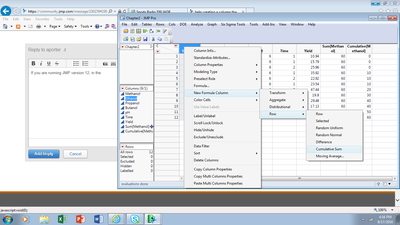Our World Statistics Day conversations have been a great reminder of how much statistics can inform our lives. Do you have an example of how statistics has made a difference in your life? Share your story with the Community!
Choose Language Hide Translation Bar
Highlighted

## help creating a column that is a cumulative sum based on other columns

I am trying to create a new column in JSL that will be an ongoing sum of the previous rows in that column.  I have a column called "Total Daily Flow"  I also have a column which marks what location the data in "total daily flow came from (call that "Site"). for example,  Say on Oct. 1, (which is specified in another column called "day of water year") i have a total daily flow of 1000, and this day happens to be day 1 of the year.  I want a column whose first row will be = 1000.  then say on day two, which will be the second row in the table, Oct. 2, there is a total daily flow of 5000, I would want the new row in the new column to be 1000 + 5000 = 6000.  then say Oct. 3 has a daily flow of 3000, so the next row will be 6000+3000 = 9000.  This is to go on for every row in my table that shares the same location name (Site) and is in the same "year" as specified by the column "day of water year" (1-365).

2 ACCEPTED SOLUTIONS

Accepted Solutions
Highlighted

## Re: help creating a column that is a cumulative sum based on other columns

hi aporter​,

until someone smarter comes up with a one liner you can try my plumbers solution:

Names Default To Here( 1 );

dt = Open( "\$SAMPLE_DATA/Big Class.jmp" );

dt << New Column( "Row", formula( :Row() ), eval formula );

dt:row << delete formula;

// assuming age is time and sex is site

dt << Sort( By( :sex, :age ), Order( Ascending, Ascending ), replace table );

// now sum as you want it

dt << New Column( "sumhightbysex",

formula( If( Row() == 1, :height, :sex == Lag( :sex, 1 ), :height + Lag( :height, 1 ), :sex != Lag( :sex, 1 ), :height, Empty() ) ), eval formula

);

dt:sumhightbysex << delete formula;

// sort to original order

dt << Sort( By( :Row ), Order( Ascending ), replace table );

// clean up

dt << delete column (row);

Highlighted

## Re: help creating a column that is a cumulative sum based on other columns

Hi Ron,

This is a great solution, thanks for posting.

The formula for the new column sumheightbysex has a slight typo. It should be

If(Row() == 1, :height, :sex == Lag(:sex, 1), :height + Lag(:sumhightbysex, 1), :sex != Lag(:sex, 1), :height, Empty())

using the value in the new column to add to the height, and not the height from previous row,  :height + Lag(:height), 1).

G'day

4 REPLIES 4
Highlighted

## Re: help creating a column that is a cumulative sum based on other columns

If you are running JMP version 12, if you right click on the column header field in the data table, the New Formula Column -> Row -> Cumulative should work without having to resort to JSL. See below:Highlighted

## Re: help creating a column that is a cumulative sum based on other columns

hi aporter​,

until someone smarter comes up with a one liner you can try my plumbers solution:

Names Default To Here( 1 );

dt = Open( "\$SAMPLE_DATA/Big Class.jmp" );

dt << New Column( "Row", formula( :Row() ), eval formula );

dt:row << delete formula;

// assuming age is time and sex is site

dt << Sort( By( :sex, :age ), Order( Ascending, Ascending ), replace table );

// now sum as you want it

dt << New Column( "sumhightbysex",

formula( If( Row() == 1, :height, :sex == Lag( :sex, 1 ), :height + Lag( :height, 1 ), :sex != Lag( :sex, 1 ), :height, Empty() ) ), eval formula

);

dt:sumhightbysex << delete formula;

// sort to original order

dt << Sort( By( :Row ), Order( Ascending ), replace table );

// clean up

dt << delete column (row);

Highlighted

## Re: help creating a column that is a cumulative sum based on other columns

Hi Ron,

This is a great solution, thanks for posting.

The formula for the new column sumheightbysex has a slight typo. It should be

If(Row() == 1, :height, :sex == Lag(:sex, 1), :height + Lag(:sumhightbysex, 1), :sex != Lag(:sex, 1), :height, Empty())

using the value in the new column to add to the height, and not the height from previous row,  :height + Lag(:height), 1).

G'day

Highlighted

## Re: help creating a column that is a cumulative sum based on other columns

Perhaps the oneliner can be based on this:

Re: Syntax: How do I create an index column by ID

it would also make it independent of sorting and maintain an active formula.

but i am not sure how to tackle it.

Best

Article Labels

There are no labels assigned to this post.